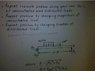# Static Equilibrium: Solving Homework Problem

• *Jim*

## Homework Statement

The problem is attached below.

## Homework Equations

Is there only 3 equations to be solved for? Sum of Forces in X and Y direction and Moment at A?

## The Attempt at a Solution

I know everything needs to be set to zero. But getting beyond this is confusing me. The distributed load does not have a specific length given, would it be multiplied by 1/2 or 1/3 of 15ft? And is the concentrated load calculated by 600 sin 60 then times the total length of 15 ft? For Ay = 502 and Ax = 7010 is what I ended up calculating but I have a feeling it is way off. Any help is welcome I am trying to understand this example problem to complete the 3 that are listed in the picture.

#### Attachments

•equilibrium.jpg
34.5 KB · Views: 326
Your problem is a bit vague. What exactly must you be solving for? The directs say "repeat problem." What is "the problem"? Some of us might be able to figure it out. It looks like a rotational equilibrium problem, but hey, I am teaching a class right now.

And what is that "600 K" at 60 degrees?

I have to replace the values for each of the 3 questions, along with Free Body Diagrams. Then put there reactions in an excel document. The 600 is 600 kips in the picture. Initially what's pictured is an example and I have to changes the values for each question. I am trying to solve the example so I understand the proper way to calculate the 3 questions.